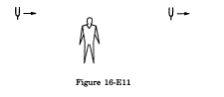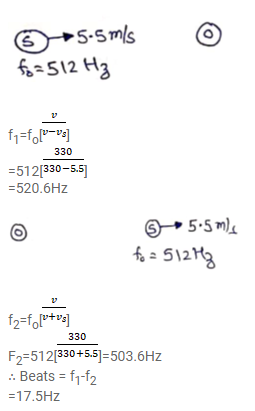# Figure shows a person standing somewhere in betweenQuestion:

Figure shows a person standing somewhere in between two identical tuning forks. each vibrating at $512 \mathrm{~Hz}$. If both the tuning forks move towards right a speed of $5.5 \mathrm{~m} \mathrm{~s}^{-1}$, find the number of beats heard by the listener. Speed of sound in air = $330 \mathrm{~m} \mathrm{~s}^{-1}$.Solution: